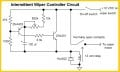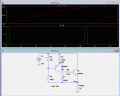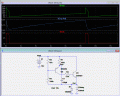# Help walking through circuit for intermittent wipers

#### Circuits123

Joined Dec 7, 2012
64
Can someone help me understand how this circuit works (attached)? I understand what all the symbols are except the triangle at the bottom. Is that a ground? Assuming it is, here's what I think I understand so far:
1. the 12v relay turns the motor on and off
2. when the relay is energized, it closes the "normally open contact" to the motor, so current flows from +12 VDC through the wire at the right of the diagram
3. the 2N4401 is a NPN transistor, meaning (I think) no current can flow from the collector to the emiter unless there's enough current applied to the base.
4. the other transistor is PNP. I assume it works the same way, but I don't understand why this is used rather than a NPN.
5. So, I think when the wiper switch is on, the only complete circuit at first is via the 47k resistor on the left.
6. Current via the 47k resistor goes to the base of the PNP transistor and turns it on, allowing current traveling via the 10k resistor to go from the collector to the emiter. The amount of current from C to E is controlled by the 500k potentiometer.
7. Once the PNP transistor is turned on, current can flow to the base of the NPN transistor and the capacitor. I don't understand what that arrangement does. I know the potentiometer controls the duration of the on/off interval for the wiper motor, but I can't figure out how.

Can someone walk me through this circuit? - Thanks#### crutschow

Joined Mar 14, 2008
24,950
At the start, the PNP base has a positive voltage from the 47k and 100k resistive divider and the capacitor is a 0V so it's base-emitter junction is reverse biased and both transistors are off.
The capacitor slowly charges from the current through the 10k resistor and the 500k pot. When the capacitor voltage reaches about 0.6V above the resistive divider voltage, the PNP's base-emitter junction becomes forward biased and the PNP starts to turn on.
The PNP collector current then turns on the NPN which energizes the relay.
The relay contacts now power the wiper motor, starting the wiper sweep.

Now because the circuit common is floating and connected to the relay coil, the common voltage will rise with the relay voltage so the voltage across the circuit becomes essentially 0V.
This discharges the capacitor, turning the relay off.
This initiates the delay for the next cycle.

Below is an LTspice simulation of the circuit.
The relay is simulated by a 75Ω resistor, typical of 12V automotive type relay resistance.
As you can see, the voltage across the capacitor (top trace) rises until it reaches about 9.4V where the circuit turns on and energizes the relay (Vout).
This drops the voltage across the circuit to zero and the capacitor then discharges until it reaches about 3V where the circuit transistors and relay turns off, starting another cycle.#### AnalogKid

Joined Aug 1, 2013
8,450
When Q1 turns on, why doesn't the Q2 emitter voltage go above V1, driven up by the bootstrap effect of C1's rapid 13V movement?

Separate from that, one of the things that helps the circuit reset at the end of each cycle s the relay coil's inductance. When Q1 turns off, the relay's inductive kick tries to create a negative voltage spike. This is coupled through the capacitor to the Q2 emitter, pulling it below the base to guarantee that the PNP is completely off. I'm not the LTSpice wiz that Wally is, but I'll see if I can demonstrate this.

Wally - what is a good simulation for an automotive relay coil?

ak

Last edited:

#### crutschow

Joined Mar 14, 2008
24,950
Here's a simulation of the circuit with the inductance of the relay and the flyback diode added.
I used this to estimate the value of the relay inductance and use a relay coil resistance of 75 ohms.
As can be seen, the bootstrap effect does raise the voltage at Q2's emitter to about 20V when the relay turns on (top trace).
The second trace is the voltage across the capacitor.
The coils inductance doesn't have a large effect on the circuit operation since the flyback diode keeps the voltage to within 0.7V of ground.#### Attachments

• 1.5 KB Views: 3
Last edited:

#### AnalogKid

Joined Aug 1, 2013
8,450
In my version I forgot the diode (!!!) and the inductive kick goes down to about -4 V.
LTS definitely is not my first language. How do you get the three separate plots?

ak

#### crutschow

Joined Mar 14, 2008
24,950
..................
LTS definitely is not my first language. How do you get the three separate plots?
Click on the Plot Window, click on Plot Settings to get the pulldown menu, select "Add Plot Plane".
Then you just click on the trace title and drag it to the plot of your choosing.

Last edited:
•AnalogKid

#### Circuits123

Joined Dec 7, 2012
64
At the start, the PNP base has a positive voltage from the 47k and 100k resistive divider and the capacitor is a 0V so it's base-emitter junction is reverse biased and both transistors are off.
The capacitor slowly charges from the current through the 10k resistor and the 500k pot. When the capacitor voltage reaches about 0.6V above the resistive divider voltage, the PNP's base-emitter junction becomes forward biased and the PNP starts to turn on.
Thanks for the explanation. That really helps. So am I correct that until the capacitor is charged, it acts like a short and the current goes to the cap. rather than through the 100k resistor? Then when the cap. is charged, the current goes via the resistor to the base of the PNP transistor?

Also, is the 500k pot. controling how long it takes for the cap. to charge?

#### crutschow

Joined Mar 14, 2008
24,950
Thanks for the explanation. That really helps. So am I correct that until the capacitor is charged, it acts like a short and the current goes to the cap. rather than through the 100k resistor? Then when the cap. is charged, the current goes via the resistor to the base of the PNP transistor?

Also, is the 500k pot. controling how long it takes for the cap. to charge?
Not quite. I think you meant to say the while the capacitor is charging, the current is going through the 100k resistor to the capacitor, not the transistor.
Then when the capacitor is charged to about 0.7V above the base voltage, the current starts going through the PNP emitter-base junction, turning on the transistor.
Yes, the 500k pot is controlling how fast the capacitor charges, and thus the time between wipes.

Last edited: